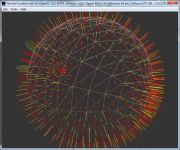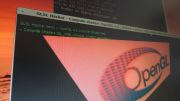Home Utilities Tutorials 3D Demos Graphics Cards Forums About

 GeeXLab
Current version: 0.29.17
>GeeXLab homepage

 FurMark
Current version: 1.21.1
>FurMark homepage

 GPU Caps Viewer
Current version: 1.44.2.1
>GPU Caps Viewer homepage

 GPU Shark
Current version: 0.16.1.0
>GPU Shark homepage

 Blogs
>JeGX's HackLab

 Geeks3D's Articles
>GPU Memory Speed Demystified

>How to Get your Multi-core CPU Busy at 100%

>How To Make a VGA Dummy Plug

>Night Vision Post Processing Filter

 PhysX FluidMark
Current version: 1.5.4
>FluidMark homepage

 TessMark
Current version: 0.3.0
>TessMark homepage

Current version: 0.3.0

 Demoniak3D
Current Version: 1.23.0
>Demoniak3D
>Libraries and Plugins
>Demos
>Codes Samples

3D Graphics Search Engine:» Back To Index

### Demoniak3D/LUA API: Models Management library

The HYP_Model library performs general operations on the models of the scene.
```HYP_Model.GetFirstSubMesh
HYP_Model.GetSubMeshByName
HYP_Model.ScaleVertices
HYP_Model.Save
HYP_Model.SetVerticesColor
HYP_Model.SetCloningMode
HYP_Model.GetNumFaces
HYP_Model.GetNumVertices
```

#### SetCloningMode

SetCloningMode allows to specify the manner the model sub meshes are cloned.
##### Syntaxe
```HYP_Model.SetCloningMode( model_name| model_id, options );
```
• model_name - [STR127] - name of the model to be cloned specified in the XML script by the name attribute.
• model_id - [INTEGER] - identifier of the model to be cloned.
• options - [INTEGER] - type of cloning:
• new clone holds its own mesh data: MESH_CLONING_NOT_SHARE_MESHDATA = 1 - default value.
• new clone shares mesh data with the base mesh: MESH_CLONING_SHARE_MESHDATA = 2.

#### GetSubMeshByName

GetSubMeshByName allows to get the model submesh identifier. This function is useful to access a submesh which is not declared in the main XML script.
##### Syntax
```mesh_id = HYP_Model.GetSubMeshByName( model_name| model_id, sub_mesh_name );
```
• model_name - [STR127] - name of the model as specified in the XML script by the "name" attribute.
• model_id - [INTEGER] - numerical identifier of the model.
• sub_mesh_name - [STR127] - name of the submesh you want to know the identifier.
• mesh_id - [INTEGER] - submesh identifier.
##### Example
```head_mesh_id = HYP_Model.GetSubMeshByName( "human", "head" );
```

#### GetFirstSubMesh

GetFirstSubMesh allows to get the identifier of the first submesh of a model.
##### Syntax
```mesh_id = HYP_Model.GetFirstSubMesh( model_name| model_id );
```
• model_name - [STR127] - name of the model as specified in the XML script by the "name" attribute.
• model_id - [INTEGER] - numerical identifier of the model.
• mesh_id - [INTEGER] - submesh identifier.
##### Example
```local id = HYP_Model.GetFirstSubMesh( "human" );
```

#### SetVerticesColor

SetVerticesColor allows to change the vertices color of all the submeshes of a model.
##### Syntax
```HYP_Model.SetVerticesColor( model_name|model_id,
new_r, new_g, new_b, new_a );
```
• model_name - [STR127] - name of the model as specified in the XML script by the "name" attribute.
• model_id - [INTEGER] - numerical identifier of the model.
• new_r - [CLAMPED_REAL] - red component of the vertices color.
• new_g - [CLAMPED_REAL] - green component of the vertices color.
• new_b - [CLAMPED_REAL] - blue component of the vertices color.
• new_a - [CLAMPED_REAL] - alpha component of the vertices color.

#### ScaleVertices

ScaleVertices allows to apply a scale factor to the vertices of each one of submeshes model. The original position of the vertices is automatically saved. This makes that when using a factor of 1.0, the vertices will take place again in their original position.
##### Syntax
```HYP_Model.ScaleVertices( model_name|model_id,
scale_factor_x, scale_factor_y, scale_factor_z );
```
• model_name - [STR127] - name of the model as specified in the XML script by the "name" attribute.
• model_id - [INTEGER] - numerical identifier of the model.
• scale_factor_x - [REAL] - X scaling factor of the vertex.
• scale_factor_y - [REAL] - Y scaling factor of the vertex.
• scale_factor_z - [REAL] - Z scaling factor of the vertex.

Load allows to load a 3d model (*.3DS, *.ASE, *.FBX, *.OBJ, *.O3MDL, etc...) and to automatically add it to the scene tree.
##### Syntax
```model_id = HYP_Model.Load( filename, loading_options );
```
• filename - [STR255] - access path relating to the file of the model to be loaded.
• model_id - [INTEGER] - numerical identifier of the model.
##### Example
```teapot_id = HYP_Model.Load( "teapot.3ds",
HYP_Object.SetPosition(teapot_id, 0.0, 50.0, -20.0 );
end;
```

#### Save

Save allows to save a model in the O3MDL format.
##### Syntax
```HYP_Model.Save( model_name|model_id, filename );
```
• model_name - [STR127] - name of the model as specified in the XML script by the "name" attribute.
• model_id - [INTEGER] - numerical identifier of the model.
• filename - [STR255] - access path relating to the file of the model to be saved.

LoadAmbientOcclusionMap allows to load a file containing the ambient occlusion data in order to make Ambient Occlusion Lighting. This XML file, called occlusion map, contains for each vertex of each submesh, the values of the bent_normal vector as well as the occlusion term.

The occlusion map creation is an offline stage because it is a time consuming process (several hours). For this reason, Hyperion is delivered with the AOTPP.exe utility (command line tool), which will generate this occlusion map.
##### Syntax
```HYP_Model.LoadAmbientOcclusionMap( model_name|model_id, filename );
```
• model_name - [STR127] - name of the model as specified in the XML script by the "name" attribute.
• model_id - [INTEGER] - numerical identifier of the model.
• filename - [STR255] - access path relating to the occlusion map.

#### GetNumFaces

GetNumFaces allows to get the number of triangular faces of all sub meshes.
##### Syntax
```nfaces = HYP_Model.GetNumFaces( model_name| model_id );
```
• model_name - [STR127] - name of the model as specified in the XML script by the "name" attribute.
• model_id - [INTEGER] - numerical identifier of the model.
• nfaces - [INTEGER] - number of faces.

#### GetNumVertices

GetNumVertices allows to get the number of vertices of all sub meshes.
##### Syntax
```nverts = HYP_Model.GetNumVertices( model_name| model_id );
```
• model_name - [STR127] - name of the model as specified in the XML script by the "name" attribute.
• model_id - [INTEGER] - numerical identifier of the model.
• nverts - [INTEGER] - number of vertices.

 GeeXLab demosGLSL - Mesh exploderPhysX 3 cloth demoNormal visualizer with GS